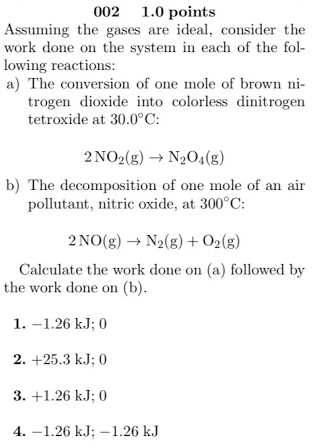# Assuming the gases are ideal, consider the work done on the system in each of the following reactions: a) The conversion of one mole of brown nitrogen dioxide into colorless dinitrogen tetroxide at 30.0°C: 2NO(g) → N2O4(g) b) The decomposition of one mole of an air pollutant, nitric oxide, at 300°C: 2NO2(g) → N2(g) + O2(g) Calculate the work done on (a) followed by the work done on (b). 1. -1.26 kJ; 0 2. +25.3 kJ; 0 3. +1.26 kJ; 0 4. -1.26 kJ; -1.26 kJ